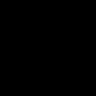# C++ program to remove all character from string except alphabets

## Removing all characters from the string except alphabets.

Removing all characters excepts alphabets means removing special characters like ‘*’, ‘?’,  ‘@’,  etc. and numeric characters like ‘1’, ‘2’, ‘3’, etc. from the input string.

input string :- 1_pre9pins34a

Output sting:- prepinsta## Algorithm:

• Initialize the variables.
• Accept the input.
• Initialize a for loop and terminate it at the end of string.
• Iterate each character through the loop.
• Remove non alphabetical characters using while loop.
• Store only alphabetical characters from the string using another for loop
• Print result.

## C++ programming code to remove all characters from string except alphabets

Run
```#include <iostream>
using namespace std;
int main()
{
//Initializing variable.
char str;
int i, j;

//Accepting input.
cout<<"Enter a string : ";
gets(str);

//Iterating each character and removing non alphabetical characters.
for(i = 0; str[i] != '\0'; ++i)
{
while (!( (str[i] >= 'a' && str[i] <= 'z') || (str[i] >= 'A' && str[i] <= 'Z') || str[i] == '\0') )
{
for(j = i; str[j] != '\0'; ++j)
{
str[j] = str[j+1];
}
str[j] = '\0';
}
}
//Printing output.
cout<<"After removing non alphabetical characters the string is :";
puts(str);
return 0;
}```
```Output:
Enter a string : *1prep_insta*
After removing non alphabetical characters the string is :prepinsta
```

## Method 2

Run
```#include <bits/stdc++.h>
using namespace std;
// function to remove characters and
// print new string
void removeSpecialCharacter(string s) {
for (int i = 0; i < s.size(); i++) {
// Finding the character whose
// ASCII value fall under this
// range
if (s[i] < 'A' || s[i] > 'Z' && s[i] < 'a' || s[i] > 'z') {
// erase function to erase
// the character
s.erase(i, 1);
i--;
}
}
cout << s;
}
// driver code
int main() {
string s = "P*re;p..ins't^a?";
removeSpecialCharacter(s);
return 0;
}```

#### Output

`Prepinsta`

### Related Banners

Get PrepInsta Prime & get Access to all 200+ courses offered by PrepInsta in One Subscription

### 6 comments on “C++ program to remove all character from string except alphabets”

•akshay

#include
using namespace std;

int main(int argc, char const *argv[])
{
string str=”P*re;p..ins’t^a?”;
int n=str.length();
string res=””;

for(int i=0; i<n; i++){
if(isdigit(str[i]))
continue;
if(isalpha(str[i]))
res+=str[i];
else
continue;
}

cout<<res;
return 0;
}0
•PrepInsta Support0
•PrepInsta Support0
•vishal

#include
#include
using namespace std;
int main(){
string str;
cout<<"enter a string"<= ‘a’ && str[i] = ‘A’ && str[i] <= 'Z'))
{
cout<<str[i];
}
}
return 0;

}0
•Pooja

//another simple solution
#include
#include

using namespace std;

int main ()
{

char ch;
cin>>ch;
int count=0;
int len=strlen(ch);
for(int i=0; i=’A’&& ch[i]=’a’&& ch[i]<='z'))
{
continue;
}
else
{
for(int j=i; j<len; j++)
{
ch[j]=ch[j+1];
}

len–;
i–;

}
}
cout<<ch;

}0
•Promit

#include
#include
using namespace std;
int main()
{
char a,b;
int i=0,j=0,k=-1;
gets(a);
for(i=0;a[i]!=’\0′;i++)
{

if(a[i]>=’a’&&a[i]=’A’&&a[i]<='Z')
{
k++;
a[k]=a[i];

}
else
{
continue;
}
}
a[k+1]='\0';
puts(a);

}1